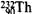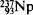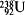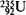Also found in: Dictionary, Medical, Wikipedia.

[¦rād·ē·ō′ak·tiv ′sir·ēz]
(nuclear physics)
A succession of nuclides, each of which transforms by radioactive disintegration into the next until a stable nuclide results. Also known as decay chain; decay family; decay series; disintegration chain; disintegration family; disintegration series; radioactive chain; radioactive decay series; series decay; transformation series.
McGraw-Hill Dictionary of Scientific & Technical Terms, 6E, Copyright © 2003 by The McGraw-Hill Companies, Inc.
The following article is from The Great Soviet Encyclopedia (1979). It might be outdated or ideologically biased.

a group of genetically related radioisotopes in which each succeeding isotope is formed as a result of the alpha or beta decay of the preceding isotope. Each radioactive series has a parent isotope, which is the one with the longest half-life. Radioactive series end in stable isotopes.

If a nucleus emits an alpha particle, its charge Z is reduced by 2 and its mass number A by 4. Upon emission of a beta particle, Z increases by 1, but A is unchanged. Thus, in each radioactive series, the mass numbers of the isotopes may be the same or may differ in multiples of 4. If the mass numbers of a given radioactive series are evenly divisible by 4, then such mass numbers may be given by the general formula 4n, where n is a whole number; on the other hand, when division by 4 leaves a remainder of 1, 2, or 3, the general formulas for the mass numbers may be written as 4n + 1, 4n + 2, and 4n + 3. These formulas are used to distinguish four radioactive series, whose parent isotopes are(4n series),(4n + 1 series),(4n + 2 series), and(4n + 3 series). The radioactive series themselves are usually named according to their parent isotopes. Thus, one speaks of the thorium, neptunium, uranium (238U), and actinouranium (235U) series. The 238U series is sometimes called the uranium-radium series (the most stable isotope of radium, 226Ra, is a member of this series). It is understood that a radioactive isotope may appear in only one specific radioactive series.

The thorium, actinouranium, and uranium-radium series (natural radioactive series) exist in nature. The reason for this is that the half-lives of 232Th (1.41 × 1010 yr), 235U (7.13 × 108 yr), and 238U (4.51 × 109 yr) are comparable to the age of the earth (several billion years) and that these isotopes have not entirely decayed. These natural radioactive series end with the isotopes of lead 208Pb, 207Pb, and 206Pb.

The half-life of 237Np is 2.14 × 106 yr. Therefore, neptunium and its radioactive series are not found in nature; these isotopes were all obtained artificially in the 1940’s and 1950’s by means of nuclear reactions. The 237Np series ends with the stable bismuth isotope 209Bi.

Each radioactive series includes both long-lived and shortlived isotopes (see Figure 1 in ). If an isotope belongs to a natural radioactive series, then it must be present in nature, even if the rate of decay of its nuclei is great. This is related to the establishment, with time, of secular equilibrium in radioactive series. The time required to attain such equilibrium in each series is equal to approximately ten half-life periods of the longest-lived intermediate member of the series. In secular equilibrium, the rate of formation of an isotope is equal to its rate of decay. Thus, the content of such an isotope remains virtually unchanged over centuries. Its content decreases at an undetectably low rate only as the parent isotope of the series decays.

The establishment of secular equilibrium in natural radioactive series explains the presence in nature of such relatively unstable radioactive chemical elements as protactinium, actinium, radium, francium, radon, astatine, and polonium. The content of each of these isotopes in nature decreases with decreasing half-life of the corresponding isotopes of the radioactive series. Thus, 1 ton of uranium in nature corresponds to only about 0.34 g of 226Ra, which has a half-life of about 1,600 yr.

Most members of natural radioactive series have special names and symbols. For example, the isotope 230Th is called ionium (symbol Io), 214Po is called radium C′ (RaC″), and 228Ra is called mesothorium 1 (MsTh1). These names arose historically even before the concept of isotopes was understood.

Some isotopes that are members of radioactive series have two modes of decay rather than one (alpha or beta decay). The nuclei of such isotopes emit alpha particles in some cases and beta particles in others. For example, 227Ac, in the actinouranium series, undergoes alpha decay in 988 out of 1,000 cases and beta decay in the remaining 12 cases. The probability in percent of each mode of decay is indicated in the figure (see above) by the numbers near the arrows corresponding to alpha and beta decay of such an isotope.

### REFERENCES

See references under .

S. S. BERDONOSOV